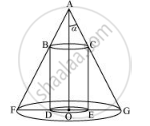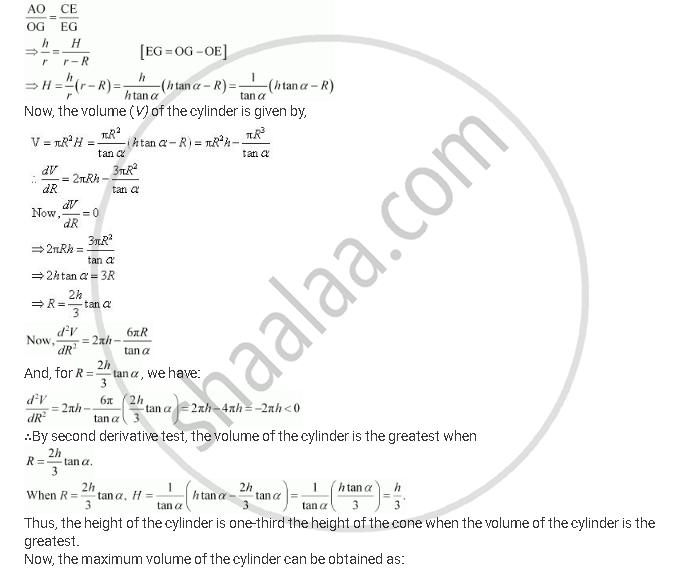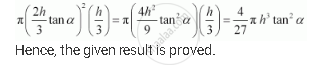# Show that Height of the Cylinder of Greatest Volume Which Can Be Inscribed in a Right Circular Cone of Height H And Semi Vertical Angle α Is One-third that of the Cone and the Greatest Volume of Cylinder is 4/27 Pih^3 Tan2α. - Mathematics

Show that height of the cylinder of greatest volume which can be inscribed in a right circular cone of height h and semi vertical angle α is one-third that of the cone and the greatest volume of cylinder is 4/27 pih^3 tan2α.

#### Solution

The given right circular cone of fixed height (h) and semi-vertical angle (α) can be drawn as:Here, a cylinder of radius R and height H is inscribed in the cone.

Then, ∠GAO = α, OG = r, OA = h, OE = R, and CE = H.

We have,

h tan α

Now, since ΔAOG is similar to ΔCEG, we have:Concept: Maximum and Minimum Values of a Function in a Closed Interval
Is there an error in this question or solution?

#### APPEARS IN

NCERT Class 12 Maths
Chapter 6 Application of Derivatives
Exercise 6.6 | Q 18 | Page 243

Share